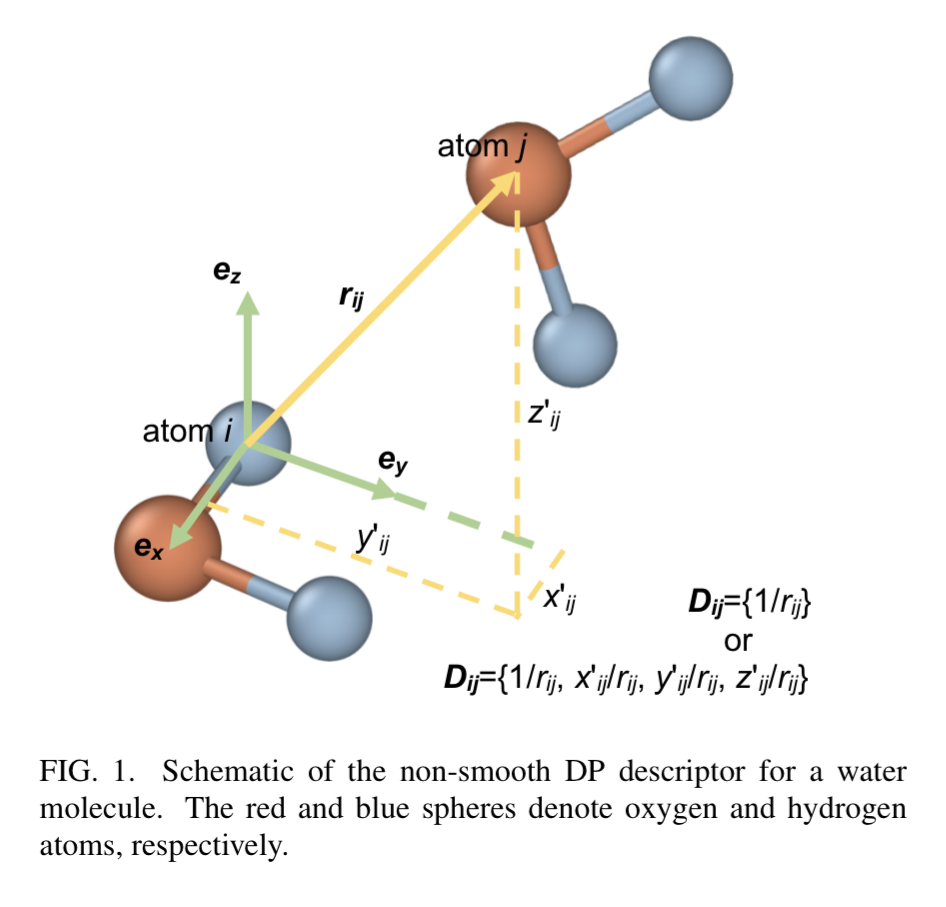# The DP descriptor

## The vanilla non-smooth DP

### The descriptor $\{\mathcal{D}_{ij}\}$

• Radial information: $1/r_{ij}$
• Distance info: $(x' / r_{ij}, y' / r_{ij}, z' / r_{ij})$.The rotation matrix \mathcal{R} that transforms the global coordinate to the local coordinate in the vanilla non-smooth DP. (Wen et al., 2022)

## The smooth DP (DP-SE)

### Overview

A simplified description of the process of constructing the energy is the following:

A more complete depiction can be seen in (Wen et al., 2022) as shown in the following figure:

### Nomenclature

Variable Description Dimension
$\mathcal{R}_i$ the environment matrix for atom $i$ $(N_i, 3)$
$\tilde{\mathcal{R}}_i$ the augmented matrix for atom $i$ $(N_i, 4)$
$\mathcal{G}_i$ the embedding matrix $(N_i, M)$
$\mathcal{D}_i$ the smooth descriptors $(M, M')$
$E_i$ the energy of atom $i$

More precisely, the map $\mathcal{G}_i \to \mathcal{R}_i$ is given by (Lu et al., 2021):

where $\mathcal{S}_{i<}$ denotes the first “$i<$” columns of $S_i$. Here, $\mathcal{S}_i$ is of dimension $(M, 4)$ and $\mathcal{S}_i$ is of dimension $(M', 4)$ where $M' < M$.

### Variants of the descriptor

The superscript enclosed in parenthesis denote the variant of the descriptor, including

Description Embedding Descriptor
Two-body embedding with radial distance between neighbouring atoms $\mathcal{G}^{(2,r)}_i$ $\mathcal{D}^{(2,r)}_i$
Two-body embedding with coordinates of the neighbour atoms $\mathcal{G}^{(2,a)}_i$ $\mathcal{D}^{(2,a)}_i$
Three-body embedding with the angle between neighbour atoms in the embedding term $\mathcal{G}^{(3)}_i$ $\mathcal{D}^{(3)}_i$

In the DeePMD-kit implementation, they are named se_e2_r, se_e2_a, and se_e3, respectively. The accuracy and resolution between the three descriptor variants are $\mathcal{D}_i^{(3)} > \mathcal{D}_i^{(2,a)} > \mathcal{D}_i^{(2,r)}$ (Wen et al., 2022).

### Implementation

The implementation details in DeePMD-kit are described in the API documentation for the following functions:

The specific shape of network (adjacent layers have same or twice the width) allows the use of ResNet (deep residual neural network) (He et al., 2015).